《金哥百变Python零基础教程1》~循环与控制

# 本章知识点 :> `循环与控制`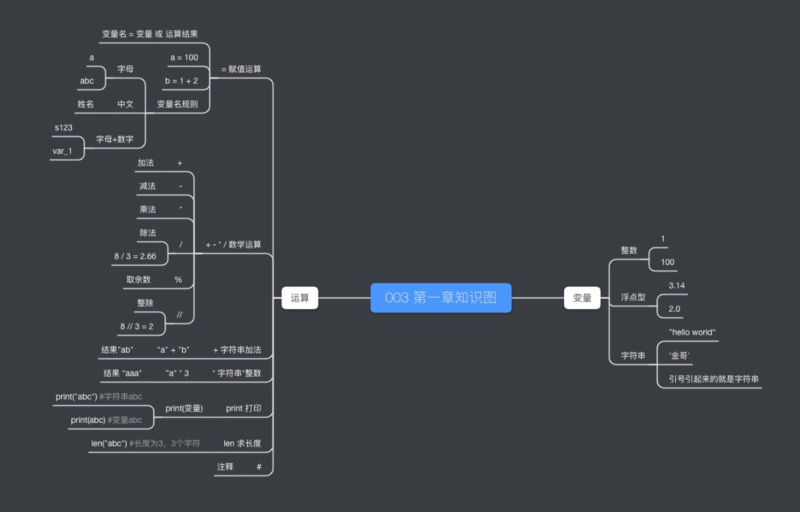# 复习：基本定律

Python编程基本能力：`可以识别Python代码里，哪些是变量，哪些是运算`

`myname = '阿金'`
`print('我是‘ + myname)`
`codeage = 20`
`print('我已编程')`
`print(codeage)`
`print('年')`

# 把几个运算符合起来比较难看懂

`codeage = 20`
`s_codeage = str(codeage)`

• str运算（学名是str函数），可以把一个整数 `codeage` 转化成字符串 `s_codeage`, 此时的 `s_cogeage`值就是 `"20"`

 类型转化 语法 例子 其他类型转字符串 str str(123) 转整型 int int("123") 转浮点型 float float("3.14") 转布尔型(后面细讲) bool bool(1)

`codeage = 20`
`print('我已编程' + str(codeage) + '年')`

`print('我已编程' + str(codeage) + '年')`

`a = ’我已编程'`
`b = str(codeage)`
`c = a + b`
`d = '年'`
`e = c + d`
`print(e)`

• 当然这里不是真的让你把这个算式写这么多步，我们只要在头脑里运行即可，其实随着我们对代码越来越熟悉，这个过程也会变得很自然
试一下 在线Python

# 比较运算

`a = 1`
`b = 2`
`b > a`

• `b > a` 表示的不是 `b 大于 a`这个结果，而是比较计算 `b 大于 a吗？`，这样说不太好理解的话，可以把代码稍微改一下

`a = 1`
`b = 2`
`c = b > a`
`print(c)`
`d = b < a`
`print(d)`

• 凡是运算都有结果，`c` 等于 `b > a` 比较计算完的结果。在这个例子里，`c` 的值为 `True`;
• `b < a` 也是一个运算，`d` 的值为 `False`;
• 在Python里，`True``False` 是布尔类型的变量，分别表示 `真``假`
• 比较运算的结果只能是 布尔类型，`b 大于 a吗？``真` (`True`); `b 小于a吗？``假`( `False`)
试一下 在线Python
• 除了 `>` `<` 还有别的比较运算
 操作符 含义 例子 == 等于 1 == 1 #True != 不等于 1 != 2 #True < 小于 1 < 2 #True > 大于 1 > 2 #False <= 小于等于 1 <= 1 #True >= 大于等于 1 >= 1 #True

• 易错点：布尔值 `True``False`的首字母是大写的，`true``false` 不是布尔型
`a = true #会报错`
`a = True #对`
• 易错点：判断两个变量相等用 `==` , 千万不要用 `=` (=是赋值运算)

` 1 == 1 #正确`
` 1 = 1 #会报错`
试一下 在线Python

• 其他数据类型也都可以比较运算

`print('jinge' == 'jinge')`
`print('abc' >= 'cda')`
`print(True == False)`
`print(1 == 1.0)`
`print(10 != 'dog')`
试一下 在线Python

• 布尔类型之间还可以进行布尔运算(不是比较）；`and` `or` `not` 运算结果还是 布尔类型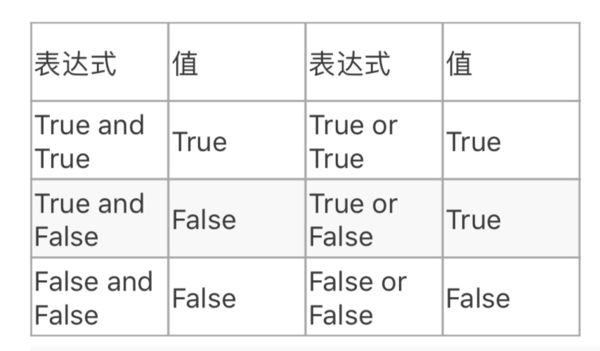• 举几个栗子：

• `print(2 > 1 and 3 > 1) #为True`
`print(20 < 50 or 20 > 50) #为True`
• 这两个表达式里，先进行比较运算，再进行了布尔运算
试一下 在线Python

# `while` 循环

`a = 0`
`a = a + 1`
`a = a + 1`
`a = a + 1`
`a = a + 1`
`print(a) #结果为4`
• 再看一个栗子：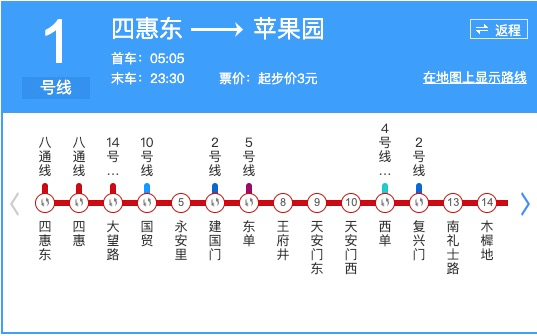`print('右走1站')`
`print('右走1站')`
`print('右走1站')`
`print('右走1站')`
`#一共走了4站`

• 上面2个程序的共同点是把 `相同的运算` 做了多遍，人话：`执行重复的运算`
• 这种情况，我们可以用 `while` 循环来写

`while` 循环的语法：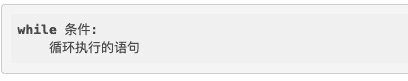• 这个语法比较复杂，我会3个方面来解释一下
• `条件` 是一个布尔运算表达式，比如 `a < 4` 表示条件是 a 小于4的时候，重复执行下面的语句
• `条件` 后面要写一个 `:` (英文的冒号), 另起一行表示循环执行的语句，要用 `四个空格` 开头

• 现在换一种图示的方法：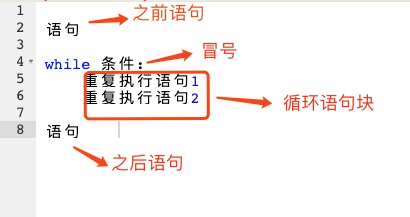• 要用4个空格把 `循环语句块` 缩进一级，表达的意思就是这块是循环内的代码

• `:` 表达的意思是，`条件` 写完了，接下来的是 `循环语句块`

• `a = a + 1` 用循环来实现一遍

• `a = 0`
`a = a + 1`
`a = a + 1`
`a = a + 1`
`a = a + 1`
`print(a) #结果为4`
• 套用语法：

• `a = 0 #之前语句`
`while a < 4:`
`a = a + 1`
`print(a) #之后语句`

试一下 在线Python

• 初学者写 `循环` 语句要有`循环思维`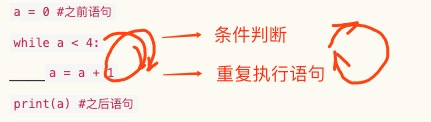• 所谓循环思维，就是每次循环不要把条件判断忘记了

• 或者我们可以换一个图来解释：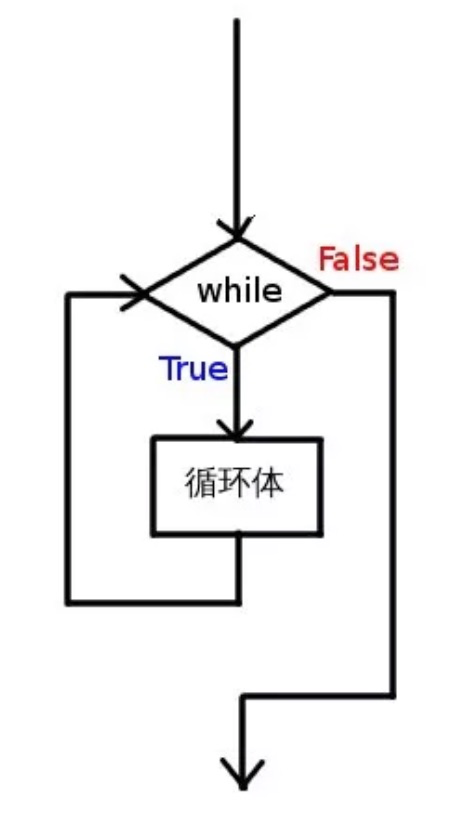• 现在改写一下 `地铁循环`

• `print('右走1站')`
`print('右走1站')`
`print('右走1站')`
`print('右走1站')`
`#一共走了4站`
• 套用循环语法

• `a = 0`
`while a < 4:`
`print('右走1站')`
`a = a + 1`

试一下 在线Python
• 易错点：在循环体内，容易把控制循环条件的变量 漏掉，比如：

• `a = 0`
`while a < 4:`
`print('右走1站')`

试一下 在线Python
• 这个写法的错误可以结合`循环流程图`看一下，我们会发现每次做 `while` 条件 `a < 4` 判断时

• `a` 的值一直是 `0`（因为没有在寻循环体改变它) 所以 `条件` 一直为 `True`
• 这样，循环会一直执行（也就是通常说的死循环）

# if 分支

• `循环` 是初学编程最难的知识点，如果理解了循环，我们再来看`if` 会轻松很多
• 我们先看看 一种循环的情况

`a = 4`
`while a < 4:`
`print("右走1站")`

• 我们读一下这段代码，会发现 `循环体` 根本没机会执行，因为 `条件` 一开始就是 `False`

`a = 0`
`while a < 1:`
`print("右走1站")`
`a = a + 1`

• 再仔细读读一下这一段循环，我们发现`循环体` 只会执行1遍，因为执行1遍之后a就为1了，`条件``False`
• 现在可以这么说，我们对 `循环` 的理解更进一步了，原来 `循环` 是可以执行 `0 -> ∞`（无穷) 次重复

# if 分支

• 对于只要执行 `0``1` 次的情况，换句话说，只要判断执行或不执行的情况，可以用 `if` 语句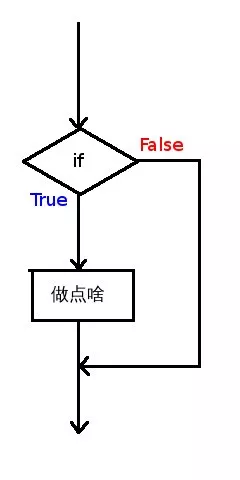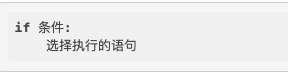• `if` （如果）后面的条件为 `True` 就执行，否则，不执行

• `:` `条件` `四个空格` `语句块` 这几个的意思和循环里是一样的
• 现在我们把例子改写一下：

`a = 0`
`if a < 1:`
`print("右走1站")`
`#其他语句`

• 可以用中文来理解为：如果(`if`) `a 小于 1`，就 `print("右走1站")`
试一下 在线Python

# 综合运用

• 我们大概又讲了20多个`简单重复` 的定式，你可以练一遍再开始我们的综合运用

`while` 循环 和 `if` 分支 没有改变程序是顺序执行的这一点，不过他们可以控制某些`语句` 执行或不执行

• 不要小看 `条件``控制` ,在这两个工具的帮助下，我们的程序才变得智能起来
• 继续上一章的问题：• 问题：我们还是简化一下1号线地铁问题，假设只为 王府井（8号站）写一个Python程序，实现路人问路，并且通过打印

`"右走1站"`
`"右走1站"`
`...`

`"左走1站"`
`"左走1站"`
`...`

• 思考下怎么写？
• 还记得 `”王府井右行乘客问路回答算法“ ` 吗？
• `start = '王府井'`
`start_num = 8`
`end = '西单'`
`end_num = 11`
`fangxiang = '右'`
`zou_num = end_num - start_num`
`print('往' + fangxiang + '走'+ str(zou_num) + '站‘)`
• 我把最后一步用 `str` 运算做了类型转化
• 因为乘客可能往左走，要完整的实现，还得写一个程序 `”王府井左行乘客问路回答算法“ `

• `start = '王府井'`
`start_num = 8`
`end = '东单'`
`end_num = 7`
`fangxiang = '左'`
`zou_num = start_num - end_num`
`print('往' + fangxiang + '走' + str(zou_num) + '站')`
• 继续，如果乘客要去的是 `"王府井"(当前站）`，我还得再写一个程序 `"王府井当站乘客问路回答算法" `

• 我去~ 这程序太不智能了？对吧

# 综合运用

• 应用 `if``while` 我们完全可以把这3个程序合成一个, 叫 `"王府井乘客问路回答算法"`
• 思路

• 这个编程思路的核心要点是把问题进行分解

• 针对这个场景，我们可以把

`"右走1站"`
`"右走1站"`
`...`

`"左走1站"`
`...`

• 命名为新运算 `指路回答法`

• 命名为新运算 `当前站指路法`
• 现在，我们假设这两个方法有现成`代码块`(熟悉这个名词吧），来整理下思路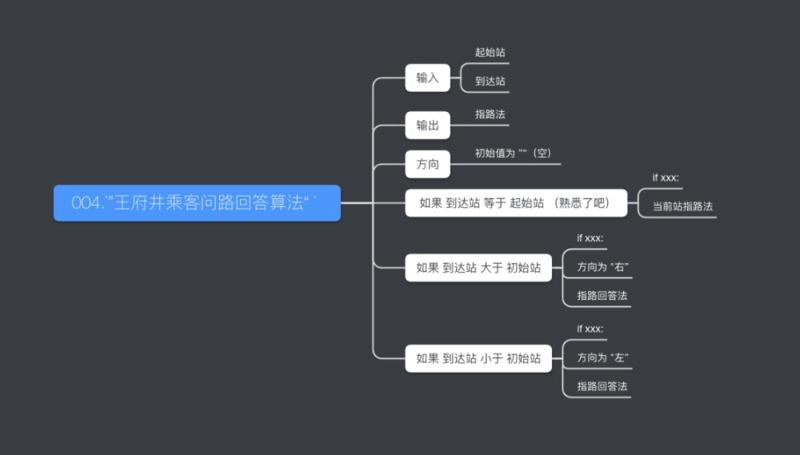• 再稍微进一步，就是主程序的代码了：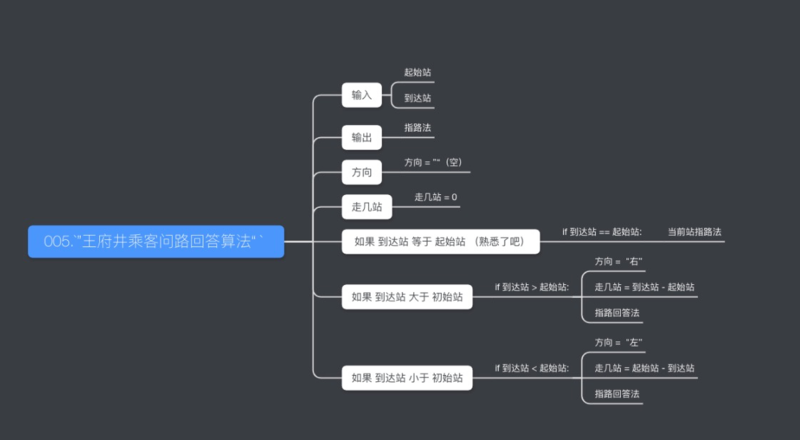`start = '王府井'`
`start_num = 8`
`end = '?' #具体执行时填写`
`end_num = ? #具体执行时填写`
`fangxiang = ''`
`zou_num = 0`
`if start_num == end_num:`
`当前站指路法`

`if start_num < end_num:`
`fangxiang = '右'`
`zou_num = end_num - start_num`
`指路回答法`

`if start_num > end_num:`
`fangxiang = '左'`
`zou_num = start_num - end_num`
`指路回答法`

• 现在我们再整理下 `当前站指路法``指路回答法`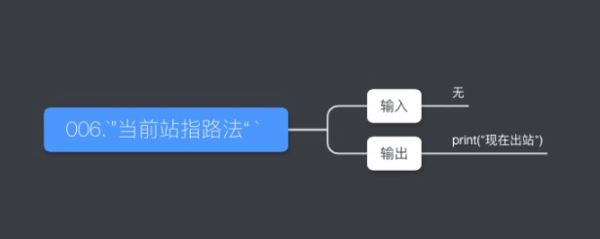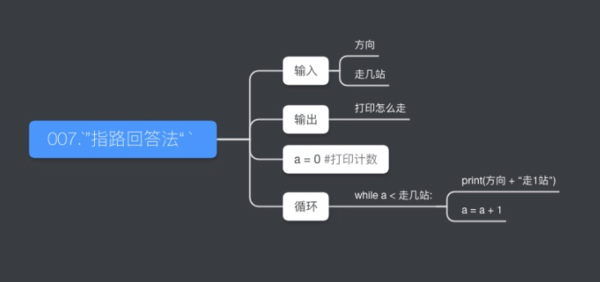• 现在可以写出完整的代码：

`start = '王府井'`
`start_num = 8`
`end = '?' #具体执行时填写`
`end_num = ? #具体执行时填写`
`fangxiang = ''`
`zou_num = 0`
`if start_num == end_num:`
`print("现在出站")`

`if start_num < end_num:`
`fangxiang = '右'`
`zou_num = end_num - start_num`
`a = 0`
`while a < zou_num:`
`print(fangxiang+'走1站') #一定要4个空格`
`a = a + 1`

`if start_num > end_num:`
`fangxiang = '左'`
`zou_num = start_num - end_num`
`a = 0`
`while a < zou_num:`
`print(fangxiang+'走1站') #一定要4个空格`
`a = a + 1`

# 下一章会先复习 `循环` 与 `if` 的知识，不用担心今天的新知识太多~

p1 知识点总结： p1总结在线看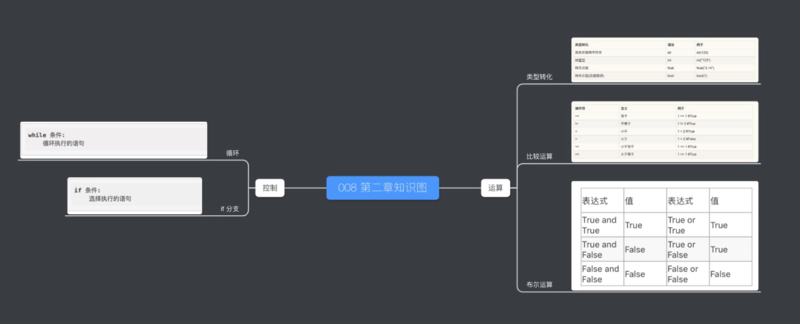Sorry but the SQLBolt exercises require a more recent browser to run.